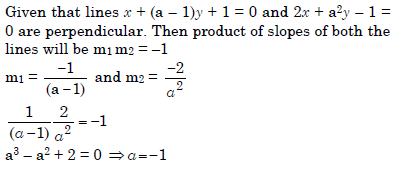## Aspire's Library

A Place for Latest Exam wise Questions, Videos, Previous Year Papers,
Study Stuff for MCA Examinations

## NIMCET Previous Year Questions (PYQs)

#### NIMCET Straight Line PYQ

NIMCET PYQ
Let a, b, c, d be no zero numbers. If the point of intersection of the line 4ax + 2ay + c = 0 & 5bx + 2by + d=0 lies in the fourth quadrant and is equidistance from the two are then

NIMCET Previous Year PYQNIMCET NIMCET 2023 PYQ

#### Solution

NIMCET PYQ
The range of values of $\theta$ in the interval $(0,\pi)$ such that the points (3, 2) and $(cos\theta ,sin\theta)$ lie on the samesides of the line x + y – 1 = 0, is

NIMCET Previous Year PYQNIMCET NIMCET 2023 PYQ

#### Solution

NIMCET PYQ
In a parallelogram ABCD, P is the midpoint of AD. Also, BP and AC intersect at Q. Then AQ : QC =

NIMCET Previous Year PYQNIMCET NIMCET 2019 PYQ

#### Solution

NIMCET PYQ
The median AD of ΔABC is bisected at E and BE is extended to meet the side AC in F. The AF : FC =

NIMCET Previous Year PYQNIMCET NIMCET 2019 PYQ

#### Solution

NIMCET PYQ
Let $a$ be the distance between the lines $−2x + y = 2$ and $2x − y = 2$, and $b$ be the distance between the lines $4x − 3y= 5$ and $6y − 8x = 1$, then

NIMCET Previous Year PYQNIMCET NIMCET 2022 PYQ

#### Solution

NIMCET PYQ
Area of the parallelogram formed by the lines y=4x, y=4x+1, x+y=0 and x+y=1

NIMCET Previous Year PYQNIMCET NIMCET 2022 PYQ

#### Solution

NIMCET PYQ
The area enclosed within the curve |x|+|y|=2 is

NIMCET Previous Year PYQNIMCET NIMCET 2022 PYQ

#### Solution

NIMCET PYQ
A straight line through the point (4, 5) is such that its intercept between the axes is bisected at A, then its equation is

NIMCET Previous Year PYQNIMCET NIMCET 2022 PYQ

#### Solution

NIMCET PYQ
The locus of the orthocentre of the triangle formed by the lines (1+p)x-py+p(1+p)=0, (1+p)(x-q)+q(1+ q)=0 and y=0 where p≠q is

NIMCET Previous Year PYQNIMCET NIMCET 2018 PYQ

#### Solution

Straight Line

NIMCET PYQ
Equation of the line perpendicular to x-2y=1 and passing through (1,1) is

NIMCET Previous Year PYQNIMCET NIMCET 2018 PYQ

#### Solution

NIMCET PYQ
If non-zero numbers a, b, c are in A.P., then the straight line ax + by + c = 0 always passes through a fixed point, then the point is

NIMCET Previous Year PYQNIMCET NIMCET 2017 PYQ

#### Solution

Since a, b and c are in A. P. 2b = a + c
a –2b + c =0
The line passes through (1, –2).

NIMCET PYQ
If the lines x + (a – 1)y + 1 = 0 and 2x + a2y – 1 = 0 are perpendicular, then the condition satisfies by a is

NIMCET Previous Year PYQNIMCET NIMCET 2017 PYQ

#### SolutionNIMCET PYQ
The lines $px+qy=1$ and $qx+py=1$ are respectively the sides AB, AC of the triangle ABC and the base BC is bisected at $(p,q)$. Equation of the median of the triangle through the vertex A is

NIMCET Previous Year PYQNIMCET NIMCET 2021 PYQ

#### NIMCET

Online Test Series,
and More.

#### NIMCET

Online Test Series,